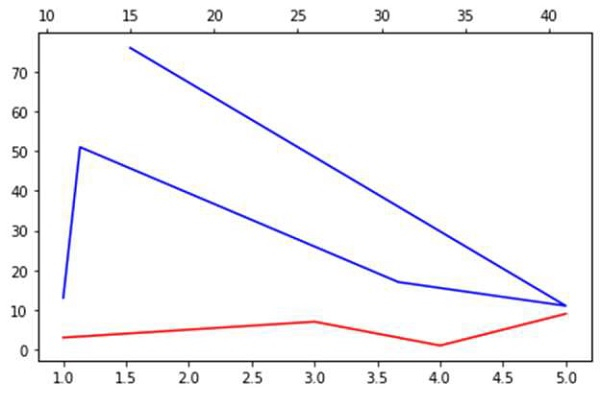# How to add a second X-axis in Matplotlib?

We can use the twiny() method to create a second X-axis. Similarly, using twinx, we can create a shared Y-axis.

## Steps

• Create fig and ax variables using subplots method, where default nrows and ncols are 1.

• Plot line with lists passed in the argument of plot() method with color="red".

• Create a twin of Axes with a shared Y-axis but independent X-axis.

• Plot the line on ax2 that is created in step 3.

• To show the figure, use plt.show() method.

## Example

import matplotlib.pyplot as plt
fig, ax1 = plt.subplots()
ax1.plot([1, 2, 3, 4, 5], [3, 5, 7, 1, 9], color='red')
ax2 = ax1.twiny()
ax2.plot([11, 12, 31, 41, 15], [13, 51, 17, 11, 76], color='blue')
fig.tight_layout()
plt.show()

## Output